# Catch up on the latest AI articles# Finally, A Model Is Now Available To Predict Multiple Material Property Value From A Single Model (MegNet)

3 main points
✔️
By using the new global quantities of states, it is possible to predict multiple materials properties with only a small amount of additional parameters
✔️ In the Pooling layer, a pooling method called Set2Set is adopted, taking into account the order of the atoms in the crystal
✔️ Visualize correlations for all elements using embedding of atoms

Graph Networks as a Universal Machine Learning Framework for Molecules and Crystals
written by (Submitted on 12 Dec 2018 (v1), last revised 28 Feb 2019 (this version, v2))

Subjects: Materials Science (cond-mat.mtrl-sci); Computational Physics (physics.comp-ph)

## Introduction

### Machine Learning in Materials Chemistry

Research into the development of new materials has been active for many years. Some of the most famous ones are catalysts, batteries, and drug discovery, which are contributing to the development of a sustainable society and industry.

In its materials chemistry, there are millions to tens of millions of candidates for the discovery of new materials, all of which are analyzed numerically based on the governing equations. However, it is difficult to do so with current computational resources. Therefore, virtual screening using machine learning is practically used as a method to narrow down the candidates.

However, there are three basic limitations (symmetries) to the physical model (governing equations) for determining the physical properties of crystals and molecules.

Firstly: Translational invariance

Second: Rotational Invariance

Thirdly: even if the same atoms are replaced, the physical properties obtained are unchanged (Permutational Invariance).

In addition to these in crystals, the

Fourth: Crystal lattices are periodic (it's easy to understand if you imagine a face-centered cubic lattice like NaCl going on forever in the X, Y, and Z directions, for example).

Fifth: symmetry in the space groups of the target crystal (the crystal lattice can be divided into 230 different space groups, each of which has its own unique symmetry).

It is a natural progression that graph neural networks satisfying these conditions will be used as a machine learning method.

However, there are three challenges to the current model.

• Many models (except for some GNN models such as SchNet) predict the properties of crystals and molecules respectively and are not very versatile.
• The amount of state which represents the characteristics of the entire system (referred to as the global state in the paper), which is necessary for the prediction of crystal properties, is not included in the model.
(e.g. temperature information is required to obtain the properties of a crystal at a certain degree of freedom, but conventional models require a model for each temperature even for a single property.)
• The biggest bottleneck for some property values is the small volume of data, for which there is no effective solution. (The number of data is directly affecting the performance.)

As a solution to all these problems, this paper proposes a GNN model called MatErial Graph Network (MEGNet).

## proposal methodology

In a traditional graph neural network, features are assigned to nodes V (nodes and vertices) and edges E (branches and edges), and training is performed by updating each feature (V, E) at the Conv layer in the GNN. (V and E are the acronyms for Vertices and Edge, respectively. )

In this paper, in addition to the features of V and E, we set up a new global state, U, which represents the features of the entire system under study. We also update u using the features of node v and edge e. The order of updating is edge E, node V, and global state U.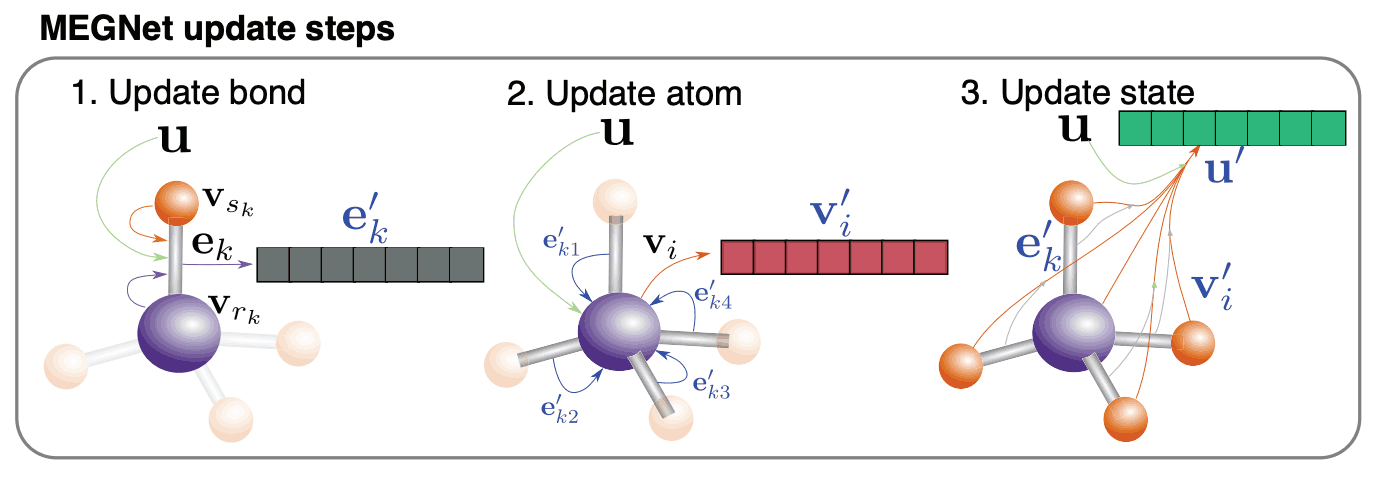The reason for updating the order of edge, node, and global state is that

The point that the Message Passing Neural Network (MPNN) was updated in the order of edges and nodes. The global state should be updated based on the updated edges and nodes. Considering these two points, I feel it makes a lot of sense.

The next section describes the model in its entirety.

The proposed model, MEGNet, consists of a multi-layered Conv layer, one Pooling layer, and a multi-layered all-coupled layer, just like general GNNs. The inputs are three features: edge features, node features, and global state, and the outputs are single physical properties.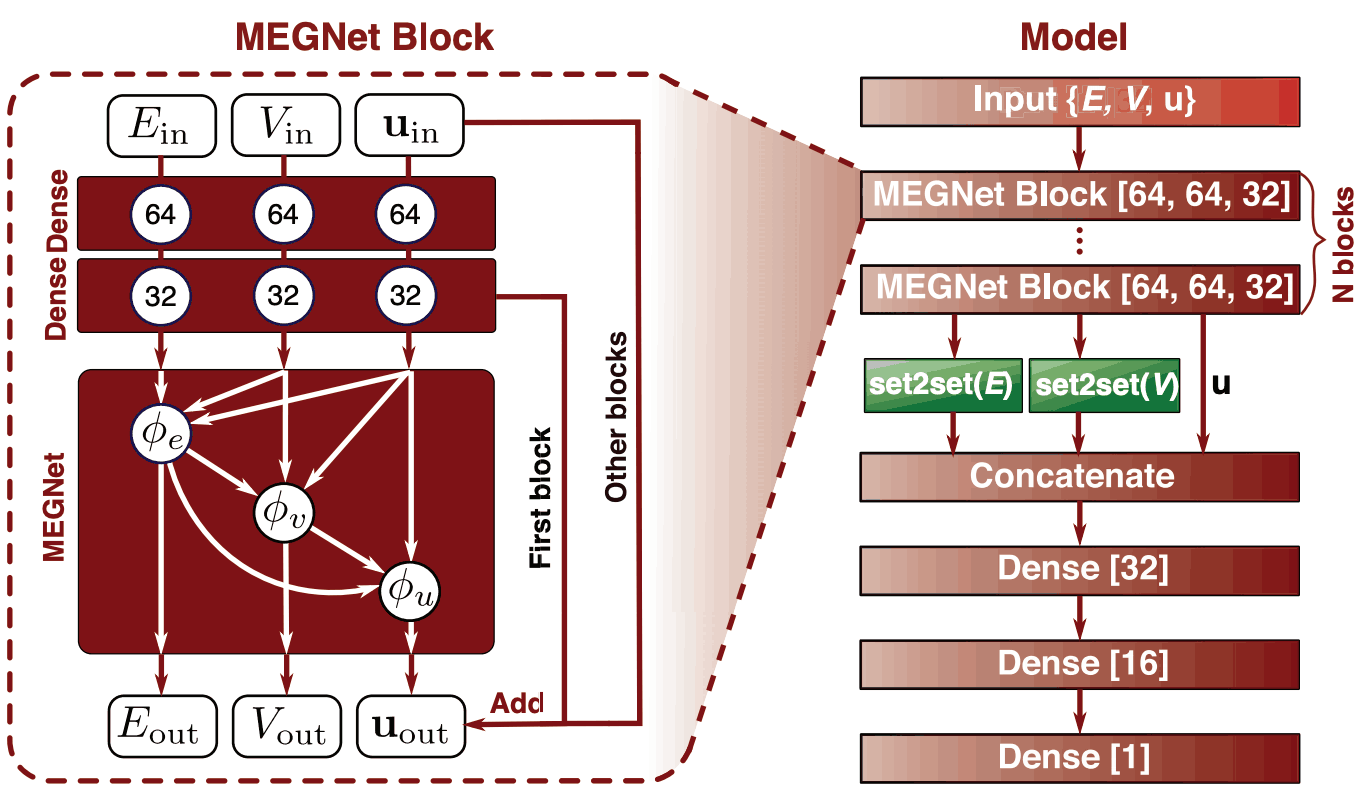Node and edge features are not identically transformed, but rather are trained with a Residual Connection (as proposed in ResNet) to avoid gradient loss in the Crystal Graph Convolutional Neural Network (CGCNN). It is used and is a common method in materials development.

The use of an all-binding layer before the MEGNet Layer is one of the small changes from the traditional GNN model.

Next, we will discuss the details of the Conv layer (MEGNet Block) and the Pooling layer (Set2Set).

How to update Edge EHow to update node VHow to update the global status UHowever, the feature update functions φ are all identical as follows.The activation function is a function called Shifted Softplus, which was used in SchNet.

For the pooling function, Sum Pooling is commonly used in GNNs, but the order information is lost; in MEGNet, we confronted this challenge with Set2Set, which uses LSTM and Attention.

### Feature Assignment

You need to assign a vector to each node, edge, and global state.

Based on previous studies, we assign the following properties to each of the molecular and crystal features.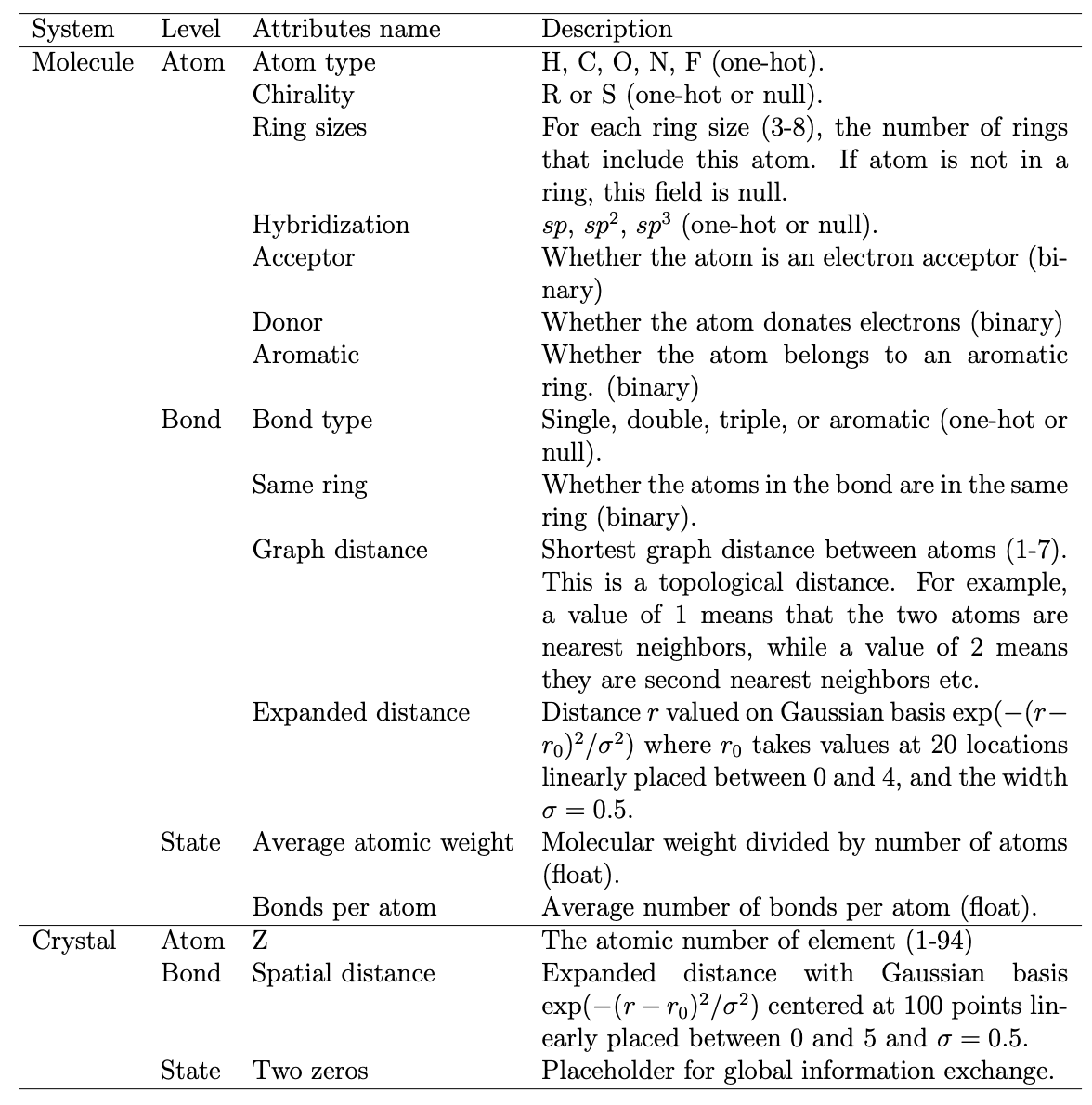We show how the four properties using global state quantities can be learned in a single model.

Its four physical properties are internal energy U0 at 0 K, internal energy U at 298 K, enthalpy H, and Gibbs' free energy of Gibbs G. The definitions of enthalpy H and Gibbs free energy G are U+PV and U+PV-TS, respectively. In order to predict multiple properties, we define the global quantity of states as three properties (T, P, S). Then the internal energy U0 at 0 K, the internal energy U at 298 K, the enthalpy H, and the free energy of Gibbs G can be expressed as (0, 0, 0), (298, 0, 0), (298, 1, 0), and (298, 1, 1), respectively. However, here, the two and three items P and S only contain information on whether they are included or not, respectively. In addition, it is possible to use four property data for one material and expect to improve the performance.

## result

Compared to SchNet, the traditional SOTA, we outperformed SchNet in the qm9(molecule) dataset by 11/13.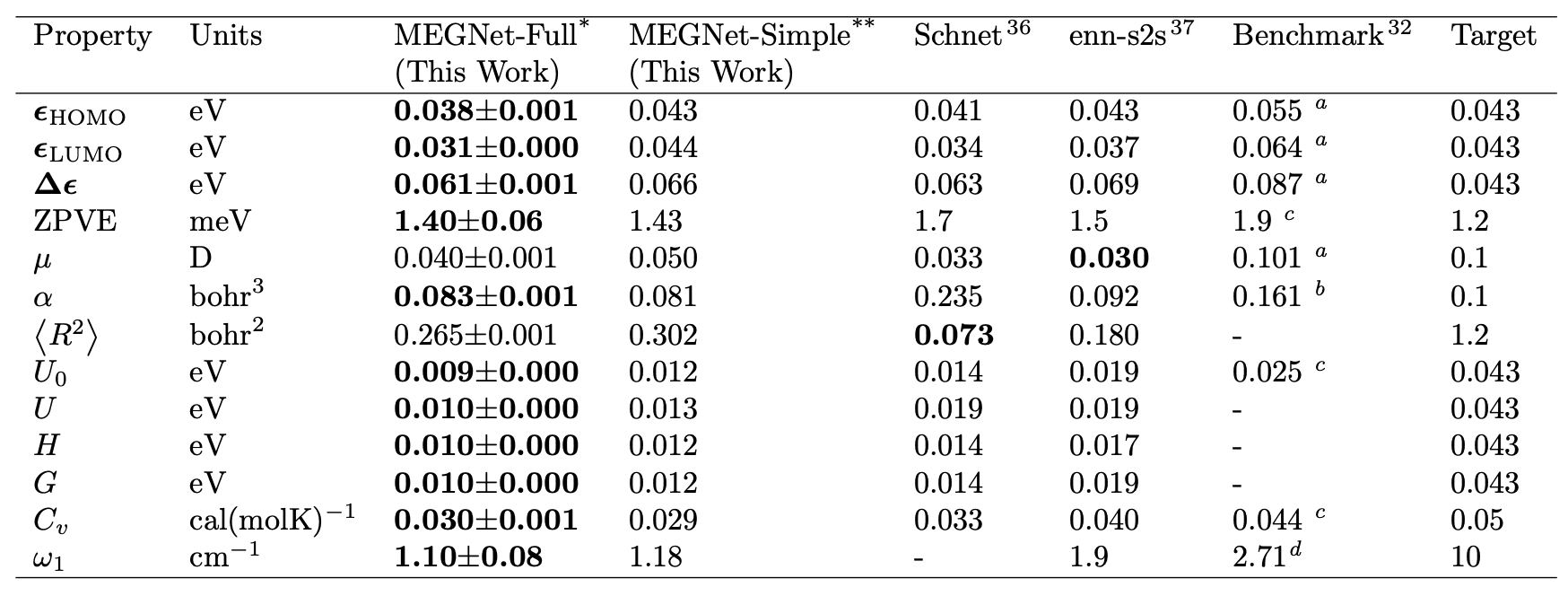They also significantly outperformed the previous model in crystals. However, it should be noted that CGCNN is a dataset as of 2017 and MEGNet is a dataset as of June 2018 and is not identical. In this regard, the author states that MEGNet is significantly better than CGCNN because of the inclusion of more complex data and still increased accuracy.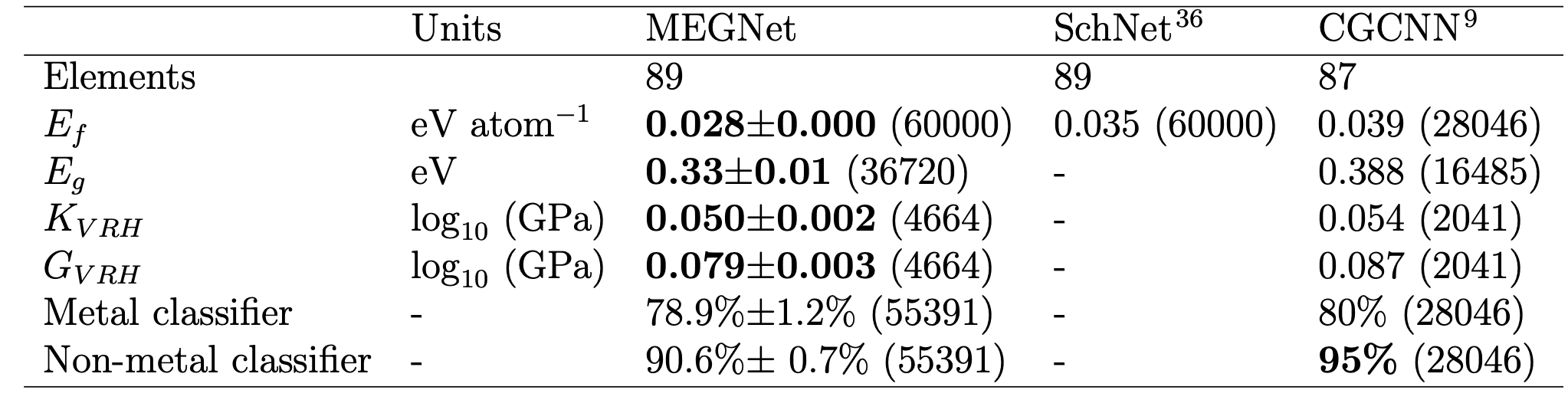The author mentions the superiority of GNNs, stating that they outperformed descriptor-based models (AFLOW-ML Model, JARVIS-ML Model) that did not use GNNs.

## Interpretation of the model

Here is a graph of Pearson's correlation coefficients taken from the embedding of atoms obtained using the formation of energy dataset. Not only are features such as alkali metals, alkaline earth metals, halogens, and lanthanides captured, but we can also see from the correlation coefficients that lanthanides such as europium and ytterbium, although they are lanthanides, exhibit similar properties to alkaline earth metals.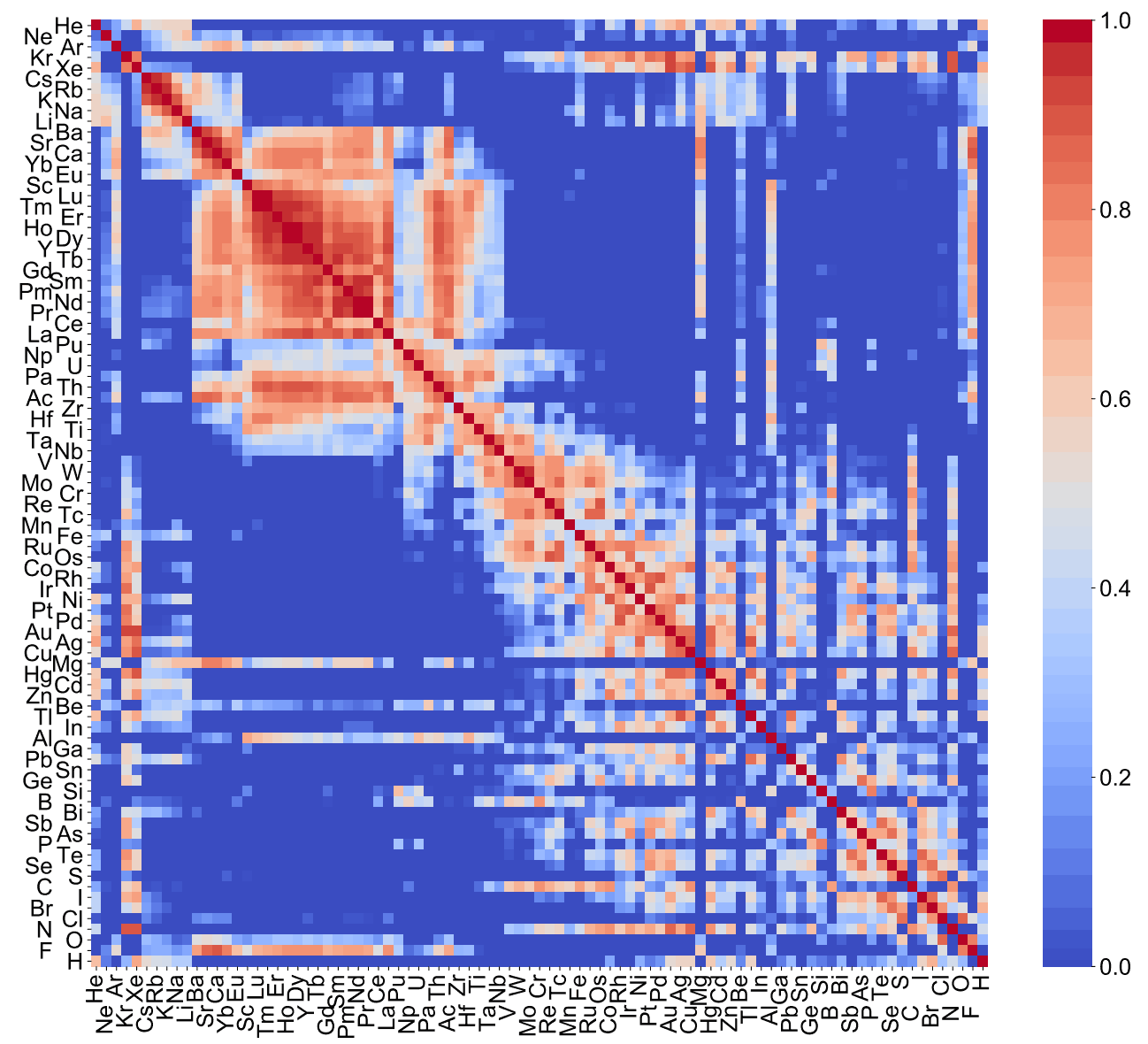The author concludes by stating, "For the small amount of data (~6500) on the elastic modulus, the transition of the atomic embedding of the formation energy confirms a sufficient improvement in performance. This is the content of this paper.

## summary

This article introduced a GNN-based property value prediction model for both molecules and crystals. The global quantities of state are interesting as an idea, and the results of the visualization of embedded atoms are an unexpected source. Let's continue to read about property prediction, which is driving research into the development of new materials that can help solve global warming and energy issues.If you have any suggestions for improvement of the content of the article,